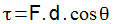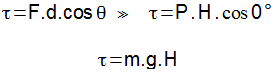Mechanics

# Conservative forces

Conservative forces are those that do work regardless of the path chosen between two distinct points.

Work (τ) is the quantity that determines how much energy has been transferred or removed from a body Its definition is made by the product of the force applied on the body and the displacement. Only forces that have the same direction as the object’s displacement do work, so the cosine of the angle between the force and the displacement must be added into the equation, so the work of a force can be defined as:Conservative forces are those that do the same work for any possible path between two points. In other words, we can say that the work of conservative forces is independent of the path taken between the starting point and the arrival point.

Note the image above in which identical objects are in free fall between two points of the same height. The only force acting on objects is weight, defined by the product of mass and acceleration due to gravity (P = mg) . Judging trajectory 2 as possible for an object to fall and starting from the mathematical definition of work, it can be determined that the work done by the weight force in any case will be the same.Note that the work is determined by the height of the fall, the straight-line distance from the point of arrival to the point of departure, and the weight of objects. Ensuring that both fall from the same height, regardless of the path taken, the job will be the same. Thus, the gravity force is a type of conservative force, for which the work is independent of the chosen path.

The forces that act on the system, transforming mechanical energy into other forms of energy, are called non-conservative forces or dissipative forces.

An example of this is the process of stopping a vehicle. When a driver applies his vehicle’s brakes, the frictional force between the brake system and the wheel is responsible for stopping the vehicle, transforming kinetic energy into sound and heat . The friction force, by transforming mechanical energy into other forms of energy, is therefore considered a dissipative force.